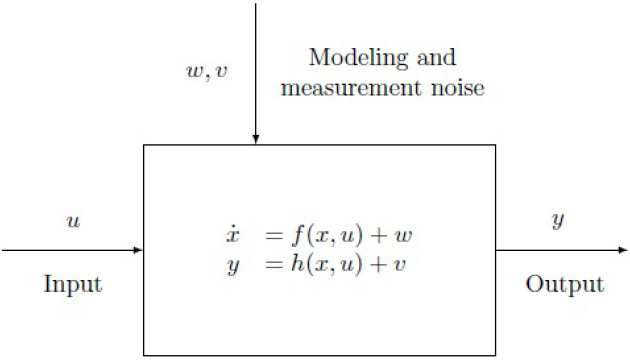# Geometric methods for nonlinear state-space system identificationSystem identification is an established scientific area which aims at  building mathematical models of dynamical systems directly from observed input-output data; such models are typically used for model-based simulation, prediction and control. System identification methods are referred to as linear and nonlinear, depending on the model sought.

Linear system identification methods form nowadays a classical subject in the field of control theory. Prediction-error methods and subspace identification methods constitute the standard approaches to the identification of linear systems. The former revolve around the minimization of a (typically nonlinear) prediction-error criterion and are widely recognized as the state of the art in single-input single-output linear system identification. The latter make use of the geometric approach to linear systems analysis and draw on ideas and algorithms arising in linear realization theory. The subspace approach gives the major advantage of converting the nonlinear optimization phase, which is the core of prediction-error methods, to a partial realization problem, which can be solved in numerically stable and efficient way. A combination of the prediction-error and subspace approaches allows to identify linear dynamical systems effectively both in a deterministic and stochastic framework.

Nonlinear system identification methods are far less understood than their linear counterpart. Tools like the neural networks, particle filters and the EM algorithm have been used in the attempt of generalizing linear prediction-error methods. While the methods considering general parameterizations for nonlinear dynamical systems almost invariably lead to computationally intractable optimization problems, the ones that postulate the model form prior to identification are often only applicable to special classes of models. On the other hand, nonlinear enhancements of subspace identification methods have only appeared for specific classes of systems, such as Hammerstein-Wiener systems, bilinear systems and linear parameter-varying systems.

The goal of this research project is twofold: first to provide a theoretical construction for the extension to nonlinear systems of linear subspace identification methods,which represent a conceptually simple and numerically reliable way to identify linear dynamical systems; second  to develop a mathematical theory of nonlinear system identification based on the differential geometric approach to nonlinear dynamical systems, which has significantly impacted on both theory and applications in many control problems.

CAP People

• Alberto Padoan - alberto.padoan13@imperial.ac.uk
• Alessandro Astolfi - a.astolfi@imperial.ac.uk